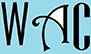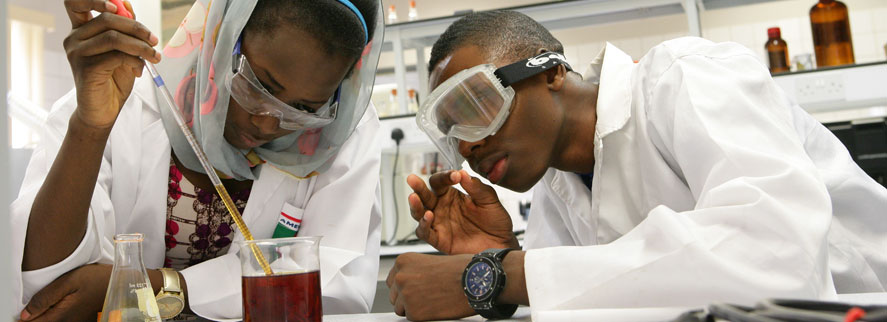## Algebra Question for Title 1 Schools: Original Cost Question?

8) Jane sold a table for \$670 which was 35% profit over the cost.  How much was the original cost of the table?

x + .35x = 670

1.35x = 670

you have to make “x” subject of formula by moving “1.35” to the other side. When you go to the other side, addition becomes subtraction, multiplication becomes division, and vice versa.

x =670/1.35 (1.35 was multiplication at the other side)

x = \$496.30